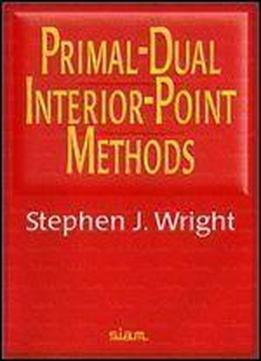# Primal-dual Interior-point Methods by Stephen J. Wright / 1987 / English / PDF

In the past decade, primal-dual algorithms have emerged as the most important and useful algorithms from the interior-point class. This book presents the major primal-dual algorithms for linear programming in straightforward terms. A thorough description of the theoretical properties of these methods is given, as are a discussion of practical and computational aspects and a summary of current software. This is an excellent, timely, and well-written work. The major primal-dual algorithms covered in this book are path-following algorithms (short- and long-step, predictor-corrector), potential-reduction algorithms, and infeasible-interior-point algorithms. A unified treatment of superlinear convergence, finite termination, and detection of infeasible problems is presented. Issues relevant to practical implementation are also discussed, including sparse linear algebra and a complete specification of Mehrotra's predictor-corrector algorithm. Also treated are extensions of primal-dual algorithms to more general problems such as monotone complementarity, semidefinite programming, and general convex programming problems.

views: 141